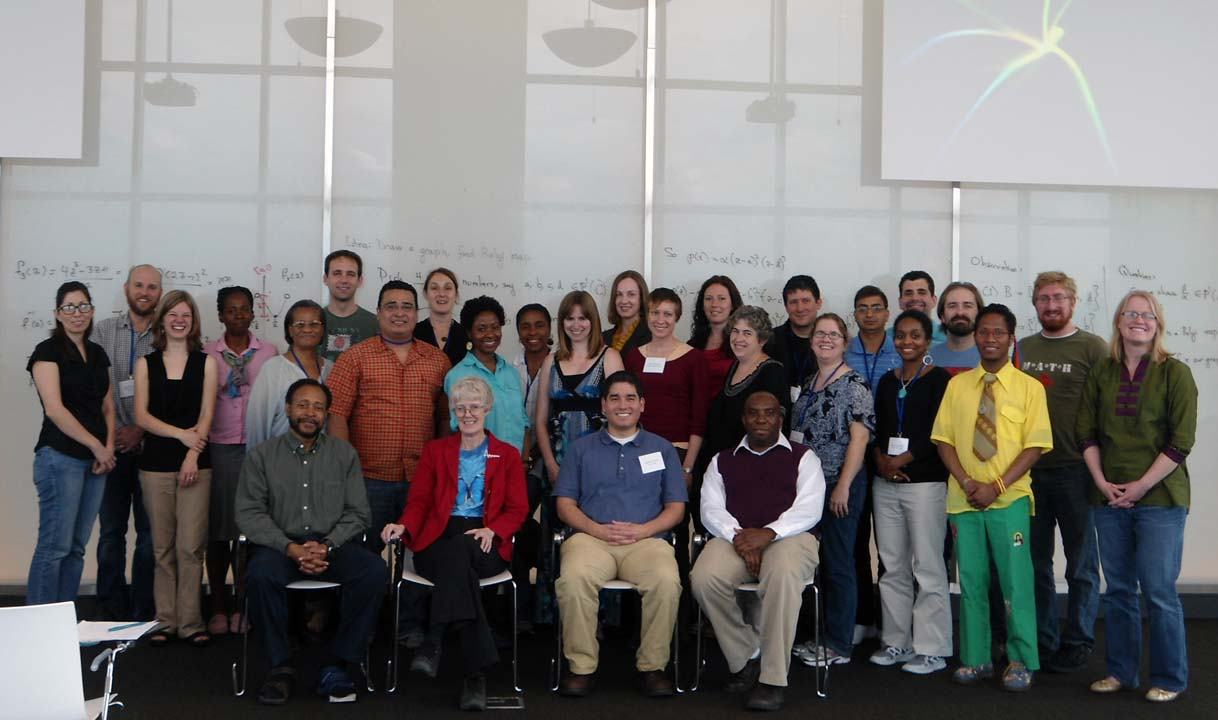# Research Experiences for Undergraduate Faculty (REUF4@ICERM)List of Participants in REUF4         more photos

Stephan's comments on undergraduate research

Trends in Undergraduate Research in the Mathematical Sciences conference in Chicago, October 26 - 28, 2012

all REUF website (requires login)

## SAGE

ISU Sage server (you will need a preset account that you get from Leslie at the workshop to use this)

Sage.org server (you can create an account here)

KAIST Groundhog Day Sage server (you can create an account here, resets every day)

Download Sage (you made need help to do this)

Quick reference to Sage

Sage online documentation and tutorial

## 1. Minimum rank, maximum nullity, zero forcing (Matrices and Graphs)

Slides related to Leslie's talk

Terminology

vector space, subspace, matrix, matrices and linear maps,matrix arithmetic, elementary row operations, (reduced) row echelon form, transpose, symmetric, nonsingular = invertible matrix, similar matrices, span, linear independence, basis, dimension, rank, range, kernel, column space, row space, determinant, properties and elementary methods of computation of determinant, eigenvalue, eigenvector, characteristic polynomial, spectrum, spectral radius, algebraic and geometric multiplicity of an eigenvalue.

Graph (simple, undirected) - note that the definition of a graph depends on the book so the first think you need to do with any book is figure out what is meant by "graph."
Subgraph, induced subgraph, path, cycle, complete graph, connected graph, connected component, graph isomorphism, planar graph, tree, adjacency matrix.

References

Any standard undergraduate linear algebra book should be fine, but it is easier to read matrix oriented ones, such as:
Howard Anton, Elementary Linear Algebra, John Wiley
David C. Lay, Linear Algebra and Its Applications, Addison Wesley
Steven .J. Leon, Linear Algebra with Applications, Prentice Hall.

The basic graph terms can be found in any elementary graph theory book or combinatorics book that has a chapter on graph theory. For example, Richard Brualdi, Introduction to Combinatorics, Prentice Hall. In the 5th edition the relevant subsection is 11.1.   Here is a PDF version of that chapter.

## 2. Prime and co-prime labeling of graphs (Graph theory)

Nate's slides

Terminology

Graph (simple, undirected) - note that the definition of a graph depends on the book so the first think you need to do with any book is figure out what is meant by "graph."
Subgraph, induced subgraph, path, cycle, complete graph, connected graph, connected component, graph isomorphism, planar graph, tree, adjacency matrix.

References

The basic terms can be found in any elementary graph theory book or combinatorics book that has a chapter on graph theory. For example, Richard Brualdi, Introduction to Combinatorics, Prentice Hall. In the 5th edition the relevant subsection is 11.1.   Here is a PDF version of that chapter.

For more detailed information, just look up the terms in the index of any of these books:
Schaum's Outline of Graph Theory: Including Hundreds of Solved Problems (Paperback) by V. Balakrishnan
Introductory Graph Theory by Gary Chartrand
Pearls in Graph Theory: A Comprehensive Introduction by Nora Hartsfield and Gerhard Ringel

## 3. Dessins d'enfants (Algebra, Graphs, Geometry)

Edray's slides

Terminology

Alternating Group, Symmetric Group, Bipartite Graph, Path Graph, Platonic Solid, Chebyshev Polynomial, Mobius Transformation

References

This short article gives an overview of Dessins d'Enfants but may be difficult to read carefully before the workshop- it may be fun to skim it:  Notices Amer. Math. Soc. 2003 Zapponi.pdf

If you get hooked by Dessins d'enfants and want to know more, here is background that will be used in the project:  background.pdf

## The basics of the real number system and limits can be found in many calculus books or introductory analysis texts.  A useful blend of calculus and number theory at the undergraduate level is: Shahriari; "Approximately Calculus" Some basic familiarity with elementary number theory may be useful, but is not strictly necessary.  Some possible references (in increasing order of sophistication) are: Pommersheim, Marks & Flapan; "Number Theory: A Lively Introduction with Proofs, Applications, and Stories" Burton; "Elementary Number Theory" Niven, Zuckerman & Montgomery; "An Introduction to the Theory of Numbers" Hardy & Wright; "An Introduction to the Theory of Numbers"

Questions or comments to hogben@aimath.org or brianna@aimath.org